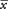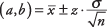## Point Estimates and Confidence Intervals

You have seen that the samplemeanis an unbiased estimate of the population mean μ. Another way to say this is thatis the best point estimate of the true value of μ. Some error is associated with this estimate, however—the true population mean may be larger or smaller than the sample mean. Instead of a point estimate, you might want to identify a range of possible values p might take, controlling the probability that μ is not lower than the lowest value in this range and not higher than the highest value. Such a range is called a confidence interval.

##### Example 1

Suppose that you want to find out the average weight of all players on the football team at Landers College. You are able to select ten players at random and weigh them. The mean weight of the sample of players is 198, so that number is your point estimate. Assume that the population standard deviation is σ = 11.50. What is a 90 percent confidence interval for the population weight, if you presume the players' weights are normally distributed?

This question is the same as asking what weight values correspond to the upper and lower limits of an area of 90 percent in the center of the distribution. You can define that area by looking up in Table 2 (in "Statistics Tables") the z-scores that correspond to probabilities of 0.05 in either end of the distribution. They are −1.65 and 1.65. You can determine the weights that correspond to these z‐scores using the following formula:The weight values for the lower and upper ends of the confidence interval are 192 and 204 (see Figure 1). A confidence interval is usually expressed by two values enclosed by parentheses, as in (192, 204). Another way to express the confidence interval is as the point estimate plus or minus a margin of error; in this case, it is 198 ± 6 pounds. You are 90 percent certain that the true population mean of football player weights is between 192 and 204 pounds.

What would happen to the confidence interval if you wanted to be 95 percent certain of it? You would have to draw the limits (ends) of the intervals closer to the tails, in order to encompass an area of 0.95 between them instead of 0.90. That would make the low value lower and the high value higher, which would make the interval wider. The width of the confidence interval is related to the confidence level, standard error, and n such that the following are true:

• The higher the percentage of confidence desired, the wider the confidence interval.

• The larger the standard error, the wider the confidence interval.

• The larger the n, the smaller the standard error, and so the narrower the confidence interval.

All other things being equal, a smaller confidence interval is always more desirable than a larger one because a smaller interval means the population parameter can be estimated more accurately.

Figure 1.The relationship between point estimate, confidence interval, and z‐score.